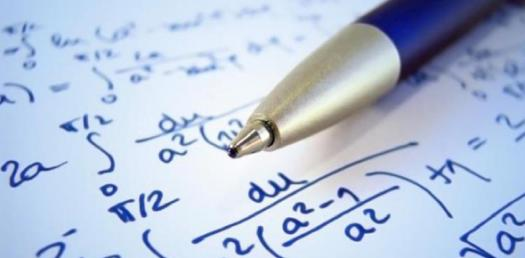# General Mathematics Trivia Quiz 2

10 Questions | Total Attempts: 331Settings.

• 1.
Add: 2 and 3/4 + 4 and 1/3
• 2.
(2 and 1/3) - (1 and 3/5)
• 3.
What is 2/5 of 45?
• 4.
What angle measure is 2/3 of a full turn?
• 5.
If an item costs \$25, find the price including a 10% sales tax. Write the dollar sign before your answer and give your answer in decimal form.
• 6.
Your meal at a diner was \$28.00. You want to leave a 20% tip for the waiter. How much money should you leave including the meal and the tip? Write the dollar sign before your answer and give it in decimal form.
• 7.
Find how many 2/3 cup measurements fit into 4 and 1/2 cups.
• 8.
Multiply: (4 and 3/5)x(2 and 1/2)
• 9.
Diane got 12 out of 15 questions correct on a recent math test. What was her percent correct? Don't forget to write % or percent after your answer.
• 10.
Charlie has 4 more than twice as many pokemon cards as Thomas. Peter has three times as many pokemon cards as Thomas. All three boys have a total of 64 cards together. How many does Peter have?
Related TopicsBack to top